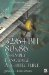Floating-Point ComparisonDo not expect the resulting values from different calculations to be identical. For example, 2.0 x 9.0 is about 18.0, and 180.0 10.0 is about18.0, but the two 18.0 values are not guaranteed to be identical.

2.0  9.0   180.0  10.0

Let us examine a range of values 10 n and compare a displacement of 0.001 versus 0.0000001.

Table 8-9: Note the single-precision loss between the 0.001 displacement as the number of digits goes up in the base number. As the base number gets larger, fewer decimal places of precision can be supported. The hexadecimal numbers in bold are where the precision was totally lost.

Base number

0.001

+0.0

+0.001

1.0

0x3F7FBE77

0x3F800000

0x3F8020C5

10.0

0x411FFBE7

0x41200000

0x41200419

100.0

0x42C7FF7D

0x42C80000

0x42C80083

1000.0

0x4479FFF0

0x447A0000

0x447A0010

10000.0

0x461C3FFF

0x461C4000

0x461C4001

100000.0

0x47C35000

0x47C35000

0x47C35000

1000000.0

0x49742400

0x49742400

0x49742400

10000000.0

0x4B189680

0x4B189680

0x4B189680

100000000.0

0x4CBEBC20

0x4CBEBC20

0x4CBEBC20

Table 8-10: This is a similar single-precision table except the displacement is between 0.0000001. Note the larger number of hexadecimal numbers in bold indicating a loss of precision.

Base number

0.0000001

+0.0

+0.0000001

1.0

0x3F7FFFFE

0x3F800000

0x3F800001

10.0

0x41200000

0x41200000

0x41200000

100.0

0x42C80000

0x42C80000

0x42C80000

1000.0

0x447A0000

0x447A0000

0x447A0000

10000.0

0x461C4000

0x461C4000

0x461C4000

100000.0

0x47C35000

0x47C35000

0x47C35000

1000000.0

0x49742400

0x49742400

0x49742400

10000000.0

0x4B189680

0x4B189680

0x4B189680

100000000.0

0x4CBEBC20

0x4CBEBC20

0x4CBEBC20

Okay, one more table for more clarity.

Table 8-11: Note that accuracy of the precision of the numbers diminishes as the number of digits increases .

Base number

+0.001

+0.002

+0.003

1.0

0x3F8020C5

0x3F804189

0x3F80624E

10.0

0x41200419

0x41200831

0x41200C4A

100.0

0x42C80083

0x42C80106

0x42C80189

1000.0

0x447A0010

0x447A0021

0x447A0031

10000.0

0x461C4001

0x461C4002

0x461C4003

100000.0

0x47C35000

0x47C35000

0x47C35000

1000000.0

0x49742400

0x49742400

0x49742400

What this means is that smaller numbers such as those that are normalized and have a numerical range from 1.0 to 1.0 allow for higher precision values, but those with larger values are inaccurate and thus not very precise. For example, the distance between 1.001 and 1.002,1.002 and 1.003, etc. is about 0x20c4 (8,388). This means that about 8,387 numbers exist between those two samples. A number with a higher digit count such as 1000.001 or 1000.002 support about 0x11(17), so only about 16 numbers exist between those two numbers. And a number around 1000000 identifies 1000000.001 and 1000000.002 as the same number. This makes for comparisons of floating-point numbers with nearly the same value very tricky. This is one of the reasons why floating-point numbers are not used for currency as they tend to lose pennies. Binary-coded decimal (BCD) and fixed-point (integer) are used instead.

So when working with normalized numbers {1.0 1.0}, a comparison algorithm with a precision slop factor (accuracy) of around0.0000001 should be utilized. When working with estimated results, a much smaller value should be used. The following function returns a Boolean true : false value to indicate that the two values are close enough to be considered the same value. Normally you would not compare two floating-point values except to see if one is greater than the other for purposes of clipping. You almost never use a comparison of the same value as shown here. It is only used in this book for purposes of comparing the results of C code to assembly code to see if you are getting results from your algorithms in the range of what you expected.

Listing 8-1: vmp_IsFEqual() Compares two single-precision floating-point values and determines if they are equivalent based upon the precision factor or if one is less than or greater than the other.bool vmp_IsFEqual(float fA, float fB, float fPrec) {      // The same so very big similar numbers or very small      // accurate numbers.   if (fA == fB) return true;          // Try with a little precision slop!   return (((fAfPrec)<=fB) && (fB<=(fA+fPrec))); }Making the call for single-precision floating-point numbers is easy:

#define SINGLE_PRECISION 0.0000001f if (!vmp_IsFEqual(f, f2, SINGLE_PRECISION))

For a fast algorithm that uses estimation for division or square roots, then merely reduces the precision to something less accurate:

#define FAST_PRECISION 0.001f

This book will discuss these fast estimate algorithms in later chapters. For vector comparisons, this book uses the following code: When dealing with quad vectors (vmp3DQVector) an alternative function is called:

Listing 8-2: Compare two {XYZ} vectors using a specified precision factor.bool vmp_IsVEqual(const vmp3DVector * const pvA,       const vmp3DVector * const pvB, float fPrec) {   ASSERT_PTR4(pvA);   // See explanation of assert macros   ASSERT_PTR4(pvB);   // later in this chapter!       if (!vmp_IsFEqual(pvA>x, pvB>x, fPrec)       !vmp_IsFEqual(pvA>y, pvB>y, fPrec)       !vmp_IsFEqual(pvA>z, pvB>z, fPrec))   {     return false;   }       return true; }Listing 8-3: Compare two {XYZW} vectors using a specified precision factor.bool vmp_IsQVEqual(const vmp3DQVector *const pvA,                    const vmp3DQVector *const pvB,                    float fPrec)

and a fourth element {.w} is tested :

!vmp_IsFEqual(pvA>w, pvB>w, fPrec)FTST FPU Test If Zero

Mnemonic

P

PII

K6

3D!

3Mx+

SSE

SSE2

A64

SSE3

E64T

FTST32/64-Bit 80x86 Assembly Language Architecture
ISBN: 1598220020
EAN: 2147483647
Year: 2003
Pages: 191

Similar book on Amazon